### Experiments are performed by a two layer NN with ReLU activation and Softmax Loss at the end. The skeleton of the model has been provided by Stanford CS231n. I do not give the codes because it is an active assignment for the students and I don't want to violate the honor code.¶

In [ ]:
# Create some toy data to check your implementations
input_size = 4
hidden_size = 10
num_classes = 3
num_inputs = 5

def init_toy_model():
model = {}
model['W1'] = np.linspace(-0.2, 0.6, num=input_size*hidden_size).reshape(input_size, hidden_size)
model['b1'] = np.linspace(-0.3, 0.7, num=hidden_size)
model['W2'] = np.linspace(-0.4, 0.1, num=hidden_size*num_classes).reshape(hidden_size, num_classes)
model['b2'] = np.linspace(-0.5, 0.9, num=num_classes)
return model

def init_toy_data():
X = np.linspace(-0.2, 0.5, num=num_inputs*input_size).reshape(num_inputs, input_size)
y = np.array([0, 1, 2, 2, 1])
return X, y

model = init_toy_model()
X, y = init_toy_data()


# Train the network¶

Here we compare naive sgd, momentum, rmsprop and rmsprop+momentum

In :
from cs231n.classifier_trainer import ClassifierTrainer

model = init_toy_model()
trainer = ClassifierTrainer()
# call the trainer to optimize the loss
# Notice that we're using sample_batches=False, so we're performing Gradient Descent (no sampled batches of data)
best_model, loss_history, _, _ = trainer.train(X, y, X, y,
model, two_layer_net,
reg=0.001,
learning_rate=1e-1, momentum=0.0, learning_rate_decay=1,
update='sgd', sample_batches=False,
num_epochs=100,
verbose=False)
print 'Final loss with vanilla SGD: %f' % (loss_history[-1], )

starting iteration  0
starting iteration  10
starting iteration  20
starting iteration  30
starting iteration  40
starting iteration  50
starting iteration  60
starting iteration  70
starting iteration  80
starting iteration  90
Final loss with vanilla SGD: 0.940686


Momentum Update

In :
model = init_toy_model()
trainer = ClassifierTrainer()
# call the trainer to optimize the loss
# Notice that we're using sample_batches=False, so we're performing Gradient Descent (no sampled batches of data)
best_model, loss_history, _, _ = trainer.train(X, y, X, y,
model, two_layer_net,
reg=0.001,
learning_rate=1e-1, momentum=0.9, learning_rate_decay=1,
update='momentum', sample_batches=False,
num_epochs=100,
verbose=False)
correct_loss = 0.494394
print 'Final loss with momentum SGD: %f' % (loss_history[-1])

starting iteration  0
starting iteration  10
starting iteration  20
starting iteration  30
starting iteration  40
starting iteration  50
starting iteration  60
starting iteration  70
starting iteration  80
starting iteration  90
Final loss with momentum SGD: 0.494394


RMSprop

In :
model = init_toy_model()
trainer = ClassifierTrainer()
# call the trainer to optimize the loss
# Notice that we're using sample_batches=False, so we're performing Gradient Descent (no sampled batches of data)
best_model, loss_history, _, _ = trainer.train(X, y, X, y,
model, two_layer_net,
reg=0.001,
learning_rate=1e-1, momentum=0.9, learning_rate_decay=1,
update='rmsprop', sample_batches=False,
num_epochs=100,
verbose=False)
correct_loss = 0.439368
print 'Final loss with RMSProp: %f' % (loss_history[-1])

starting iteration  0
starting iteration  10
starting iteration  20
starting iteration  30
starting iteration  40
starting iteration  50
starting iteration  60
starting iteration  70
starting iteration  80
starting iteration  90
Final loss with RMSProp: 0.439368


RMS+Momentum best of the this naive experiment

In :
model = init_toy_model()
trainer = ClassifierTrainer()
# call the trainer to optimize the loss
# Notice that we're using sample_batches=False, so we're performing Gradient Descent (no sampled batches of data)
best_model, loss_history, _, _ = trainer.train(X, y, X, y,
model, two_layer_net,
reg=0.001,
learning_rate=1e-1, momentum=0.9, learning_rate_decay=1,
update='rmsprop+momentum', sample_batches=False,
num_epochs=100,
verbose=False)
correct_loss = 0.439368
print 'Final loss with RMSProp+momentum: %f' % (loss_history[-1])

starting iteration  0
starting iteration  10
starting iteration  20
starting iteration  30
starting iteration  40
starting iteration  50
starting iteration  60
starting iteration  70
starting iteration  80
starting iteration  90
Final loss with RMSProp+momentum: 0.435519


In :
model = init_toy_model()
trainer = ClassifierTrainer()
# call the trainer to optimize the loss
# Notice that we're using sample_batches=False, so we're performing Gradient Descent (no sampled batches of data)
best_model, loss_history, _, _ = trainer.train(X, y, X, y,
model, two_layer_net,
reg=0.001,
learning_rate=1e-1, momentum=0.9, learning_rate_decay=1,
num_epochs=100,
verbose=False)
correct_loss = 0.439368

Final loss with Adagrad: 0.643385


RESULT: Even in a very simple toy dataset results are so demanding for the favor of RMSprop and Momentum against SGD. Best performance is observed by RMSprop+Momentum

In :
from cs231n.data_utils import load_CIFAR10

def get_CIFAR10_data(num_training=49000, num_validation=1000, num_test=1000):
"""
Load the CIFAR-10 dataset from disk and perform preprocessing to prepare
it for the two-layer neural net classifier. These are the same steps as
we used for the SVM, but condensed to a single function.
"""
# Load the raw CIFAR-10 data
cifar10_dir = 'cs231n/datasets/cifar-10-batches-py'
X_train, y_train, X_test, y_test = load_CIFAR10(cifar10_dir)

# Subsample the data
mask = range(num_training, num_training + num_validation)

# Normalize the data: subtract the mean image
mean_image = np.mean(X_train, axis=0)
X_train -= mean_image
X_val -= mean_image
X_test -= mean_image

# Reshape data to rows
X_train = X_train.reshape(num_training, -1)
X_val = X_val.reshape(num_validation, -1)
X_test = X_test.reshape(num_test, -1)

return X_train, y_train, X_val, y_val, X_test, y_test

# Invoke the above function to get our data.
X_train, y_train, X_val, y_val, X_test, y_test = get_CIFAR10_data()
print 'Train data shape: ', X_train.shape
print 'Train labels shape: ', y_train.shape
print 'Validation data shape: ', X_val.shape
print 'Validation labels shape: ', y_val.shape
print 'Test data shape: ', X_test.shape
print 'Test labels shape: ', y_test.shape

Train data shape:  (49000, 3072)
Train labels shape:  (49000,)
Validation data shape:  (1000, 3072)
Validation labels shape:  (1000,)
Test data shape:  (1000, 3072)
Test labels shape:  (1000,)


# Train a network¶

Train this simple model with different update rules.

In :
# use SGD
from cs231n.classifiers.neural_net import init_two_layer_model

model = init_two_layer_model(32*32*3, 50, 10) # input size, hidden size, number of classes
trainer = ClassifierTrainer()
best_model1, loss_history1, train_acc1, val_acc1 = trainer.train(X_train, y_train, X_val, y_val,
model, two_layer_net,
num_epochs=20, reg=1.0,
update = 'sgd',
momentum=0.9, learning_rate_decay = 0.95,
learning_rate=1e-5, verbose=True)

Finished epoch 0 / 20: cost 2.302593, train: 0.120000, val 0.158000, lr 1.000000e-05
Finished epoch 1 / 20: cost 2.302591, train: 0.129000, val 0.130000, lr 9.500000e-06
Finished epoch 2 / 20: cost 2.302589, train: 0.139000, val 0.174000, lr 9.025000e-06
Finished epoch 3 / 20: cost 2.302574, train: 0.166000, val 0.177000, lr 8.573750e-06
Finished epoch 4 / 20: cost 2.302539, train: 0.170000, val 0.193000, lr 8.145063e-06
Finished epoch 5 / 20: cost 2.302430, train: 0.188000, val 0.199000, lr 7.737809e-06
Finished epoch 6 / 20: cost 2.302224, train: 0.195000, val 0.193000, lr 7.350919e-06
Finished epoch 7 / 20: cost 2.301709, train: 0.177000, val 0.181000, lr 6.983373e-06
Finished epoch 8 / 20: cost 2.300819, train: 0.165000, val 0.179000, lr 6.634204e-06
Finished epoch 9 / 20: cost 2.296373, train: 0.151000, val 0.181000, lr 6.302494e-06
Finished epoch 10 / 20: cost 2.290793, train: 0.169000, val 0.197000, lr 5.987369e-06
Finished epoch 11 / 20: cost 2.284688, train: 0.184000, val 0.187000, lr 5.688001e-06
Finished epoch 12 / 20: cost 2.244895, train: 0.184000, val 0.193000, lr 5.403601e-06
Finished epoch 13 / 20: cost 2.196050, train: 0.156000, val 0.180000, lr 5.133421e-06
Finished epoch 14 / 20: cost 2.254494, train: 0.135000, val 0.183000, lr 4.876750e-06
Finished epoch 15 / 20: cost 2.185508, train: 0.196000, val 0.185000, lr 4.632912e-06
Finished epoch 16 / 20: cost 2.192414, train: 0.163000, val 0.186000, lr 4.401267e-06
Finished epoch 17 / 20: cost 2.227328, train: 0.179000, val 0.188000, lr 4.181203e-06
Finished epoch 18 / 20: cost 2.073986, train: 0.181000, val 0.188000, lr 3.972143e-06
Finished epoch 19 / 20: cost 2.080279, train: 0.186000, val 0.190000, lr 3.773536e-06
Finished epoch 20 / 20: cost 2.142814, train: 0.198000, val 0.199000, lr 3.584859e-06
finished optimization. best validation accuracy: 0.199000

In :
# use Momentum
from cs231n.classifiers.neural_net import init_two_layer_model

model = init_two_layer_model(32*32*3, 50, 10) # input size, hidden size, number of classes
trainer = ClassifierTrainer()
best_model2, loss_history2, train_acc2, val_acc2 = trainer.train(X_train, y_train, X_val, y_val,
model, two_layer_net,
num_epochs=20, reg=1.0,
update = 'momentum',
momentum=0.9, learning_rate_decay = 0.95,
learning_rate=1e-5, verbose=True)

Finished epoch 0 / 20: cost 2.302593, train: 0.110000, val 0.109000, lr 1.000000e-05
Finished epoch 1 / 20: cost 2.273101, train: 0.133000, val 0.154000, lr 9.500000e-06
Finished epoch 2 / 20: cost 2.057101, train: 0.201000, val 0.241000, lr 9.025000e-06
Finished epoch 3 / 20: cost 1.888748, train: 0.311000, val 0.288000, lr 8.573750e-06
Finished epoch 4 / 20: cost 1.839703, train: 0.344000, val 0.339000, lr 8.145063e-06
Finished epoch 5 / 20: cost 1.942659, train: 0.333000, val 0.366000, lr 7.737809e-06
Finished epoch 6 / 20: cost 1.847249, train: 0.361000, val 0.389000, lr 7.350919e-06
Finished epoch 7 / 20: cost 1.742119, train: 0.407000, val 0.391000, lr 6.983373e-06
Finished epoch 8 / 20: cost 1.523142, train: 0.392000, val 0.397000, lr 6.634204e-06
Finished epoch 9 / 20: cost 1.710355, train: 0.411000, val 0.411000, lr 6.302494e-06
Finished epoch 10 / 20: cost 1.675986, train: 0.426000, val 0.417000, lr 5.987369e-06
Finished epoch 11 / 20: cost 1.759964, train: 0.449000, val 0.433000, lr 5.688001e-06
Finished epoch 12 / 20: cost 1.400295, train: 0.445000, val 0.430000, lr 5.403601e-06
Finished epoch 13 / 20: cost 1.473600, train: 0.441000, val 0.430000, lr 5.133421e-06
Finished epoch 14 / 20: cost 1.665528, train: 0.437000, val 0.447000, lr 4.876750e-06
Finished epoch 15 / 20: cost 1.608525, train: 0.471000, val 0.445000, lr 4.632912e-06
Finished epoch 16 / 20: cost 1.420895, train: 0.454000, val 0.450000, lr 4.401267e-06
Finished epoch 17 / 20: cost 1.560292, train: 0.472000, val 0.444000, lr 4.181203e-06
Finished epoch 18 / 20: cost 1.585418, train: 0.450000, val 0.449000, lr 3.972143e-06
Finished epoch 19 / 20: cost 1.623252, train: 0.503000, val 0.445000, lr 3.773536e-06
Finished epoch 20 / 20: cost 1.554016, train: 0.476000, val 0.454000, lr 3.584859e-06
finished optimization. best validation accuracy: 0.454000

In :
# use RMSprop
from cs231n.classifiers.neural_net import init_two_layer_model

model = init_two_layer_model(32*32*3, 50, 10) # input size, hidden size, number of classes
trainer = ClassifierTrainer()
best_model3, loss_history3, train_acc3, val_acc3 = trainer.train(X_train, y_train, X_val, y_val,
model, two_layer_net,
num_epochs=20, reg=1.0,
update = 'rmsprop',
momentum=0.9, learning_rate_decay = 0.95,
learning_rate=1e-5, verbose=True)

Finished epoch 0 / 20: cost 2.302593, train: 0.102000, val 0.098000, lr 1.000000e-05
Finished epoch 1 / 20: cost 1.948840, train: 0.356000, val 0.329000, lr 9.500000e-06
Finished epoch 2 / 20: cost 1.933427, train: 0.383000, val 0.359000, lr 9.025000e-06
Finished epoch 3 / 20: cost 1.866497, train: 0.390000, val 0.395000, lr 8.573750e-06
Finished epoch 4 / 20: cost 1.748220, train: 0.420000, val 0.414000, lr 8.145063e-06
Finished epoch 5 / 20: cost 1.647285, train: 0.417000, val 0.427000, lr 7.737809e-06
Finished epoch 6 / 20: cost 1.636752, train: 0.406000, val 0.438000, lr 7.350919e-06
Finished epoch 7 / 20: cost 1.683645, train: 0.433000, val 0.439000, lr 6.983373e-06
Finished epoch 8 / 20: cost 1.727223, train: 0.455000, val 0.444000, lr 6.634204e-06
Finished epoch 9 / 20: cost 1.771403, train: 0.446000, val 0.454000, lr 6.302494e-06
Finished epoch 10 / 20: cost 1.662157, train: 0.484000, val 0.450000, lr 5.987369e-06
Finished epoch 11 / 20: cost 1.783750, train: 0.451000, val 0.451000, lr 5.688001e-06
Finished epoch 12 / 20: cost 1.572829, train: 0.455000, val 0.459000, lr 5.403601e-06
Finished epoch 13 / 20: cost 1.539926, train: 0.457000, val 0.459000, lr 5.133421e-06
Finished epoch 14 / 20: cost 1.699544, train: 0.439000, val 0.458000, lr 4.876750e-06
Finished epoch 15 / 20: cost 1.600255, train: 0.443000, val 0.463000, lr 4.632912e-06
Finished epoch 16 / 20: cost 1.619370, train: 0.468000, val 0.464000, lr 4.401267e-06
Finished epoch 17 / 20: cost 1.571197, train: 0.476000, val 0.464000, lr 4.181203e-06
Finished epoch 18 / 20: cost 1.608766, train: 0.468000, val 0.469000, lr 3.972143e-06
Finished epoch 19 / 20: cost 1.630492, train: 0.484000, val 0.477000, lr 3.773536e-06
Finished epoch 20 / 20: cost 1.481858, train: 0.489000, val 0.470000, lr 3.584859e-06
finished optimization. best validation accuracy: 0.477000

In :
# use RMSprop+Momentum
from cs231n.classifiers.neural_net import init_two_layer_model

model = init_two_layer_model(32*32*3, 50, 10) # input size, hidden size, number of classes
trainer = ClassifierTrainer()
best_model4, loss_history4, train_acc4, val_acc4 = trainer.train(X_train, y_train, X_val, y_val,
model, two_layer_net,
num_epochs=20, reg=1.0,
update = 'rmsprop+momentum',
momentum=0.9, learning_rate_decay = 0.95,
learning_rate=1e-5, verbose=True)

Finished epoch 0 / 20: cost 2.302593, train: 0.166000, val 0.165000, lr 1.000000e-05
Finished epoch 1 / 20: cost 1.800040, train: 0.373000, val 0.390000, lr 9.500000e-06
Finished epoch 2 / 20: cost 1.636812, train: 0.459000, val 0.437000, lr 9.025000e-06
Finished epoch 3 / 20: cost 1.609279, train: 0.472000, val 0.450000, lr 8.573750e-06
Finished epoch 4 / 20: cost 1.540035, train: 0.467000, val 0.451000, lr 8.145063e-06
Finished epoch 5 / 20: cost 1.507733, train: 0.487000, val 0.460000, lr 7.737809e-06
Finished epoch 6 / 20: cost 1.642292, train: 0.518000, val 0.473000, lr 7.350919e-06
Finished epoch 7 / 20: cost 1.497452, train: 0.504000, val 0.468000, lr 6.983373e-06
Finished epoch 8 / 20: cost 1.533577, train: 0.502000, val 0.473000, lr 6.634204e-06
Finished epoch 9 / 20: cost 1.442068, train: 0.482000, val 0.464000, lr 6.302494e-06
Finished epoch 10 / 20: cost 1.549564, train: 0.486000, val 0.473000, lr 5.987369e-06
Finished epoch 11 / 20: cost 1.494527, train: 0.502000, val 0.471000, lr 5.688001e-06
Finished epoch 12 / 20: cost 1.462458, train: 0.495000, val 0.483000, lr 5.403601e-06
Finished epoch 13 / 20: cost 1.515679, train: 0.543000, val 0.480000, lr 5.133421e-06
Finished epoch 14 / 20: cost 1.510962, train: 0.525000, val 0.485000, lr 4.876750e-06
Finished epoch 15 / 20: cost 1.541044, train: 0.508000, val 0.492000, lr 4.632912e-06
Finished epoch 16 / 20: cost 1.577317, train: 0.536000, val 0.493000, lr 4.401267e-06
Finished epoch 17 / 20: cost 1.525123, train: 0.538000, val 0.491000, lr 4.181203e-06
Finished epoch 18 / 20: cost 1.351778, train: 0.552000, val 0.503000, lr 3.972143e-06
Finished epoch 19 / 20: cost 1.590443, train: 0.536000, val 0.499000, lr 3.773536e-06
Finished epoch 20 / 20: cost 1.448961, train: 0.530000, val 0.507000, lr 3.584859e-06
finished optimization. best validation accuracy: 0.507000

In :
# AdaGrad
# use RMSprop+Momentum
from cs231n.classifiers.neural_net import init_two_layer_model

model = init_two_layer_model(32*32*3, 50, 10) # input size, hidden size, number of classes
trainer = ClassifierTrainer()
best_model5, loss_history5, train_acc5, val_acc5 = trainer.train(X_train, y_train, X_val, y_val,
model, two_layer_net,
num_epochs=20, reg=1.0,
momentum=0.9, learning_rate_decay = 0.95,
learning_rate=0.01, verbose=True)

Finished epoch 0 / 20: cost 2.302593, train: 0.117000, val 0.108000, lr 1.000000e-02
Finished epoch 1 / 20: cost 1.976317, train: 0.375000, val 0.393000, lr 9.500000e-03
Finished epoch 2 / 20: cost 1.819465, train: 0.396000, val 0.360000, lr 9.025000e-03
Finished epoch 3 / 20: cost 1.946780, train: 0.393000, val 0.396000, lr 8.573750e-03
Finished epoch 4 / 20: cost 1.948320, train: 0.429000, val 0.388000, lr 8.145062e-03
Finished epoch 5 / 20: cost 1.817160, train: 0.445000, val 0.463000, lr 7.737809e-03
Finished epoch 6 / 20: cost 1.781161, train: 0.433000, val 0.433000, lr 7.350919e-03
Finished epoch 7 / 20: cost 1.726162, train: 0.437000, val 0.431000, lr 6.983373e-03
Finished epoch 8 / 20: cost 1.945575, train: 0.467000, val 0.438000, lr 6.634204e-03
Finished epoch 9 / 20: cost 1.717542, train: 0.463000, val 0.468000, lr 6.302494e-03
Finished epoch 10 / 20: cost 1.653255, train: 0.472000, val 0.443000, lr 5.987369e-03
Finished epoch 11 / 20: cost 1.757400, train: 0.487000, val 0.448000, lr 5.688001e-03
Finished epoch 12 / 20: cost 1.612132, train: 0.487000, val 0.470000, lr 5.403601e-03
Finished epoch 13 / 20: cost 1.575829, train: 0.462000, val 0.453000, lr 5.133421e-03
Finished epoch 14 / 20: cost 1.739385, train: 0.465000, val 0.471000, lr 4.876750e-03
Finished epoch 15 / 20: cost 1.649168, train: 0.497000, val 0.461000, lr 4.632912e-03
Finished epoch 16 / 20: cost 1.844438, train: 0.482000, val 0.483000, lr 4.401267e-03
Finished epoch 17 / 20: cost 1.755178, train: 0.502000, val 0.466000, lr 4.181203e-03
Finished epoch 18 / 20: cost 1.623927, train: 0.494000, val 0.476000, lr 3.972143e-03
Finished epoch 19 / 20: cost 1.760897, train: 0.502000, val 0.474000, lr 3.773536e-03
Finished epoch 20 / 20: cost 1.795621, train: 0.501000, val 0.469000, lr 3.584859e-03
finished optimization. best validation accuracy: 0.483000


RESULT: In a real dataset perfomance differences are more significant. SGD gets 0.18 and we can scale ti to up to 0.46 by RMSprop+Momentum with the smae number of iterations.

## Look at the results¶

SGD results ---

Plots show that SGD takes so much time to reduce the loss and it only achives 0.19 accuracy at validation set. In addition, train and validation accuracies are very dispersed at many segments of the learning. I think SGD is not very reliable by these observations.

In :
# Plot the loss function and train / validation accuracies
plt.subplot(2, 1, 1)
plt.plot(loss_history1)
plt.title('Loss history')
plt.xlabel('Iteration')
plt.ylabel('Loss')

plt.subplot(2, 1, 2)
plt.plot(train_acc1)
plt.plot(val_acc1)
plt.legend(['Training accuracy', 'Validation accuracy'], loc='lower right')
plt.xlabel('Epoch')
plt.ylabel('Clasification accuracy')

Out:
<matplotlib.text.Text at 0x7fbb51db12d0>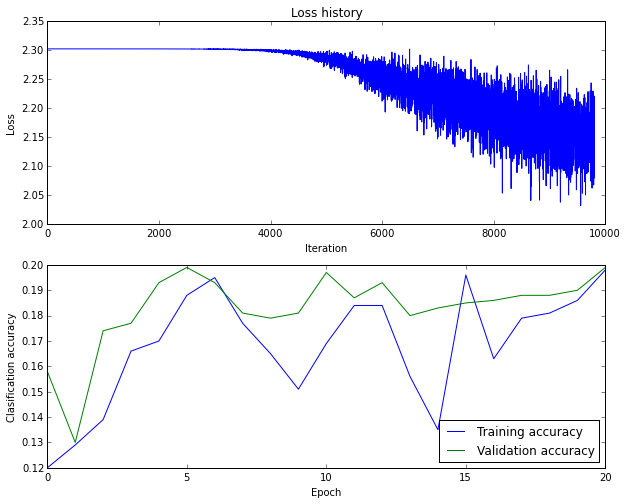Momentum Results ---

Better than SGD. However, still it needs a set of epochs to find a way to the minima. It is observed through the stationary Loss at the begining. Validation and Train accurcies much more correlated as well.

In :
# Plot the loss function and train / validation accuracies
plt.subplot(2, 1, 1)
plt.plot(loss_history2)
plt.title('Loss history')
plt.xlabel('Iteration')
plt.ylabel('Loss')

plt.subplot(2, 1, 2)
plt.plot(train_acc2)
plt.plot(val_acc2)
plt.legend(['Training accuracy', 'Validation accuracy'], loc='lower right')
plt.xlabel('Epoch')
plt.ylabel('Clasification accuracy')

Out:
<matplotlib.text.Text at 0x7fbb51f9d890>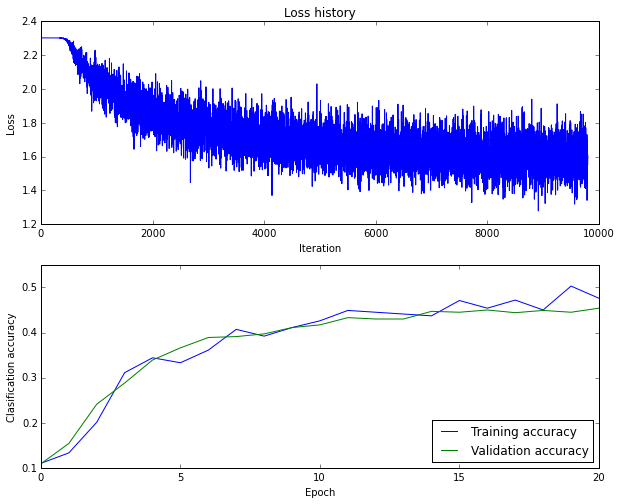RMSprop results

Main difference here is the convergende of the algorithm compared to others. You can see a very declined slope at he beginning of the Loss value. It starts to optimize very quickly. Also, accuracy is peaked from the begining of the training.

In :
# Plot the loss function and train / validation accuracies
plt.subplot(2, 1, 1)
plt.plot(loss_history3)
plt.title('Loss history')
plt.xlabel('Iteration')
plt.ylabel('Loss')

plt.subplot(2, 1, 2)
plt.plot(train_acc3)
plt.plot(val_acc3)
plt.legend(['Training accuracy', 'Validation accuracy'], loc='lower right')
plt.xlabel('Epoch')
plt.ylabel('Clasification accuracy')

Out:
<matplotlib.text.Text at 0x7fbb51d82a10>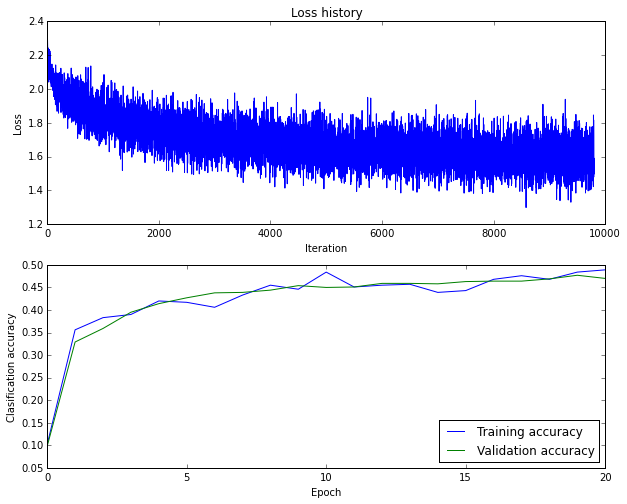RMS+Momentum Observations

Much better convergence and best performance. It peaks the validation accuracy almost at the 5th epoch and we see only a little improvement there after.

In :
# Plot the loss function and train / validation accuracies
plt.subplot(2, 1, 1)
plt.plot(loss_history4)
plt.title('Loss history')
plt.xlabel('Iteration')
plt.ylabel('Loss')

plt.subplot(2, 1, 2)
plt.plot(train_acc4)
plt.plot(val_acc4)
plt.legend(['Training accuracy', 'Validation accuracy'], loc='lower right')
plt.xlabel('Epoch')
plt.ylabel('Clasification accuracy')

Out:
<matplotlib.text.Text at 0x7fbb515bbb90>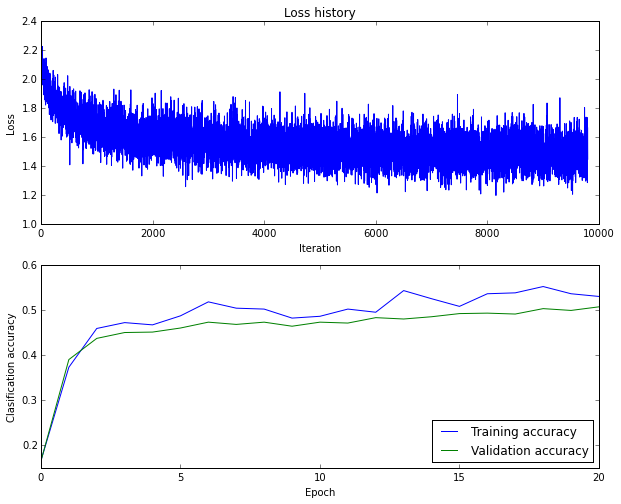AdaGrad is known as a optimization method that is robust to learning rate choice andeven you give a initial learning rate value, it finds a way to tune it to a optimal value in the couse of training, at least theoretically.

I used much greater learning rate for AdaGrad traning since it cannot improve the accuracy or the loss value otherwise.

One improtant behaviour of AdaGrad is , it needs a initial period to tune the learning to a good value. Therfore, we observe a non-decreasing even wildly increasing Loss value initially but it functions well at the end. It takes the second best accuracy as well after RMSprop+Momentum

In :
# Plot the loss function and train / validation accuracies
plt.subplot(2, 1, 1)
plt.plot(loss_history5[100:])
plt.title('Loss history -- after 100th iteration')
plt.xlabel('Iteration')
plt.ylabel('Loss')

plt.subplot(2, 1, 2)
plt.plot(train_acc5)
plt.plot(val_acc5)
plt.legend(['Training accuracy', 'Validation accuracy'], loc='lower right')
plt.xlabel('Epoch')
plt.ylabel('Clasification accuracy')

Out:
<matplotlib.text.Text at 0x7fbb51116290>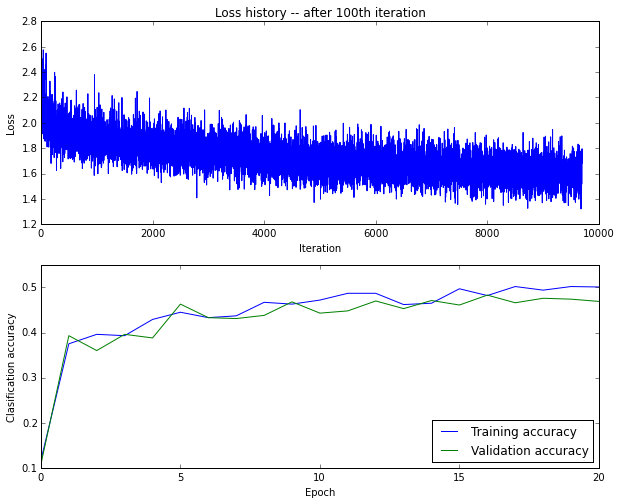AdaGrad updates takes some epochs to tune learning rate. I printed loss values for first 50 epochs to see this effect.

In :
loss_history5[0:50]

Out:
[2.3025928278904635,
75.934736079622795,
92.182474572629786,
100.5019278755542,
84.844181635490045,
49.409391098629875,
43.328290141355289,
35.325688200166425,
25.519854900507241,
21.356402138599851,
26.218600967478551,
31.59622812204384,
26.22040589643569,
23.227517734307192,
17.767912373878527,
16.157541492684935,
12.608468084163864,
12.321226053503693,
13.24122535188368,
12.928515216653592,
10.796928215446016,
9.1984105449830302,
11.298781826011448,
10.804694477862794,
12.568968109093376,
10.56363563705977,
7.9977924016860049,
7.34338664646741,
6.6929796435282283,
7.1677274578861159,
7.2066434970512532,
6.7933009055358866,
6.7913307562240677,
6.6829018897838459,
7.0043970733245429,
7.3813129930707051,
6.4100443076342426,
5.7477095369608451,
7.3967044022923663,
5.8068102116770044,
6.1110745504385751,
5.4339376326257485,
5.3695441754045357,
4.6965492787415659,
5.7784881299439856,
5.4864936711432595,
4.4452802054476042,
4.7502021504025134,
5.2173199263390639,
3.9212560040704405]

### Conclusion¶

Despite the simplicity of the both Momentum and RMSprop tricks, performance improvement is very high compared to naive SGD. Also from the above figures, you can see the problems that I've been told in my blog post http://bit.ly/1FGKb4K. If we look at the loss changes of SGD it is very unstable and after we apply Momentum it is stabilized relatively. Then implication of RMSprop makes things better and modest.

Another fact is the convergence rates of the methods. As you can see RMSprop+Momentum reaches the very solid values after only the first epoch.

I add AdaGrad to experiments lately. As I stated above, if you like to see good values in a short time AdaGrad is not the choice but in some way or another it concludes a good model, if you are patient enough.

The accuracy values of train and validation are also more correlated as we improve the SGD with the additional tricks. Of course, I didn't suppose that this model is the best and you can see that we can increase the number of learning epochs or make the model larger since there is no gap between train and validation accuracies yet after 5th epoch.

As a side note, this notebook and the blog post is inspired as I was working on Stanford CS231n assignment2. Therefore I did not provide any replicable code for the sake of honor code.